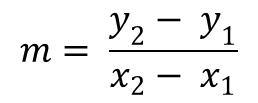# PA Chapter 5 Vocabulary Flashcards

Set Details Share
created 8 days ago by beccacook
8 views
updated 7 days ago by beccacook
Page to share:
Embed this setcancel
COPY
code changes based on your size selection
Size:
X

1

Coordinate Plane

Formed by the intersection of two number lines, the horizontal axis and vertical axis.

2

x-axis

The horizontal axis on a coordinate plane.

3

y-axis

The vertical axis on a coordinate plane.

4

The four regions into which the x-and y-axis separate the coordinate plane.

5

Origin

The point at which the x-and y-axis intersect on the coordinate plane; (0, 0).

6

Ordered Pair

A set of numbers, or coordinates, written in the form (x, y).

7

x-coordinate

The x-value of an ordered pair, represent the horizontal placement of the point.

8

y-coordinate

The y-value of an ordered pair, represents the vertical placement of the point.

9

Discrete graph

A graph that consists of points that are not connected.

10

Continuous graph

A function graphed with a line or smooth curve.

11

Relation

A set of ordered pairs.

12

Domain

The set of x-values of the ordered pairs in a relation.

13

Range

The set of y-values of the ordered pairs in a relation.

14

Function

A relation in which each element of the domain is paired with exactly one element of the range.

15

Independent Variable

The variable in a function with a value that is subject to choice.

16

Dependent Variable

The variable in a relation with a value that depends on the value of the independent variable.

17

Vertical Line Test

If any vertical line drawn on the graph of a relation passes through no more than one point on the graph for each value of x in the domain, then the relation is a function.

18

Rate of Change

A change in one quantity with respect to another quanitity.

19

Slope

The ratio of the rise, or vertical change, to the run, or horizontal change.

20

Positive Slope

A line that is slant, and is inclined upwards when observed from left to right.

21

Negative Slope

The slope of a line that is sloping downwards as we are moving from left to right.

22

Zero Slope

A line parallel to the x-axis of the coordinate system.

23

Undefined Slope

The slope of a vertical line.

24

Slope Formula25

Slope-Intercept Form

A linear equation in the form y = mx + b, where m is the slope and b is the y-intercept.

26

Standard Form

The equation is Ax + By = C, where A > 0, A and B are not both zero, and A, B, and C are integers with a greatest common factor of 1.

27

Linear Function

f(x) = y = mx + b

28

Proportional Relationship

Relationships between two variables where their ratios are equivalent.

29

Constant of Variation

The constant (unchanged) ratio of two variable quantities.

30

Direct Variation

A relationship between two variables in which one is a constant multiple of the other.